# Question Solved1 AnswerHow is the answer to part ci) Horizontal axis: R/(T^2) , where T is the time to complete one revolution Vertical axis: tan0 1. This question is a long free-response question. Show your work for each part of the question. Motor ie L String R M Block In the apparatus shown above, one end of a string of length L is attached to a block of mass M and the other end is connected to the axle of a motor that rotates, causing the block to move in a circle of radius R a constant speed vt such that the string makes an angle with the vertical. A student wants to use the apparatus to make measurements and create a graph that can be used to estimate the acceleration g due to gravity at the surface of Earth. The student can adjust the motor to achieve different tangential speeds of the block. (b) Using the experimental apparatus shown above and the available equipment, design an experimental procedure the student could use to estimate the acceleration g due to gravity at the surface of Earth. i. In the table below, list the quantities and associated symbols that would be measured in the experiment and the equipment used to measure them. Also list the equipment that would be used to measure each quantity. You do not need to fill in every row. If you need additional rows, you may add them to the space just below the table. Quantity to be Measured Symbol for Quantity length of string L radius R Time for block to make I rev. T Equipment for Measurement meter stick meterstick Stopwatch peed ii. Describe an experimental procedure to estimate the acceleration g due to gravity at the surface of Earth using the apparatus. Give enough detail so that another student could replicate the experiment. As needed, include a diagram of the experimental setup. Assume equipment usually found in a school physics laboratory is available. for the object The speed of the motor should be set so that the object revolves around it's circular path at a constant tangential speed *A stopwatch can be used to measure the time it takes to make I complete revolution around its circular path * Meterstick can be used to measure both the radius of the circular path and length of string. * by relating the rodius of the circular path and length of string with the sine of the angle * Experiment can be measured for 5 different tangential speeds that cover Sino- as wide a ronge as possible using the available equipement * Angle can be found R I о (c) The student wants to analyze data collected from the experiment by creating a linear graph that can be used estimate the acceleration g due to gravity at the surface of Earth. i. State what quantity should be plotted on the horizontal axis and what quantity should be plotted on the vertica axis to produce the linear graph. Horizontal axis: Vertical axis: -How is the answer to part ci) Horizontal axis: R/(T^2) , where T is the time to complete one revolution Vertical axis: tan0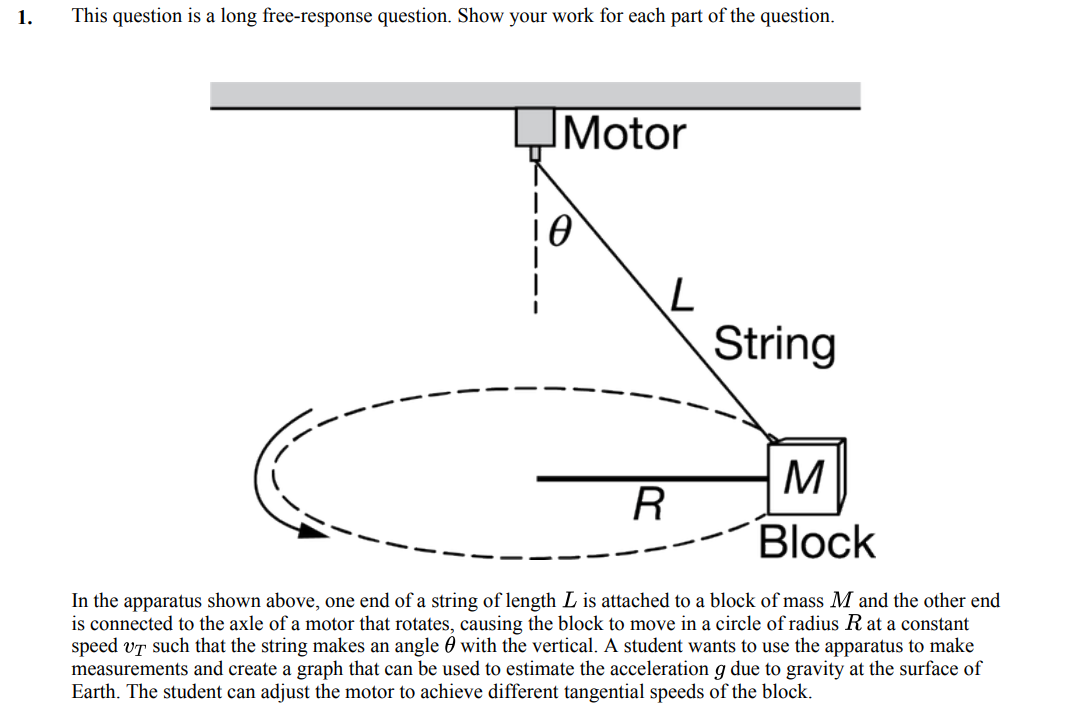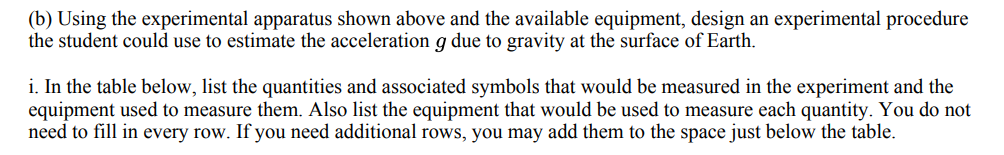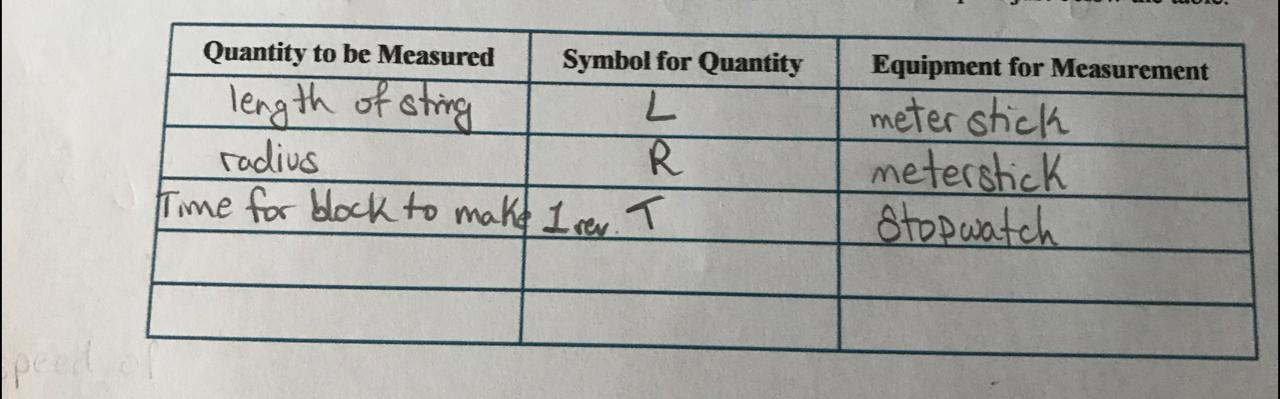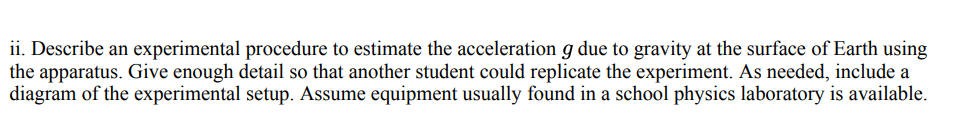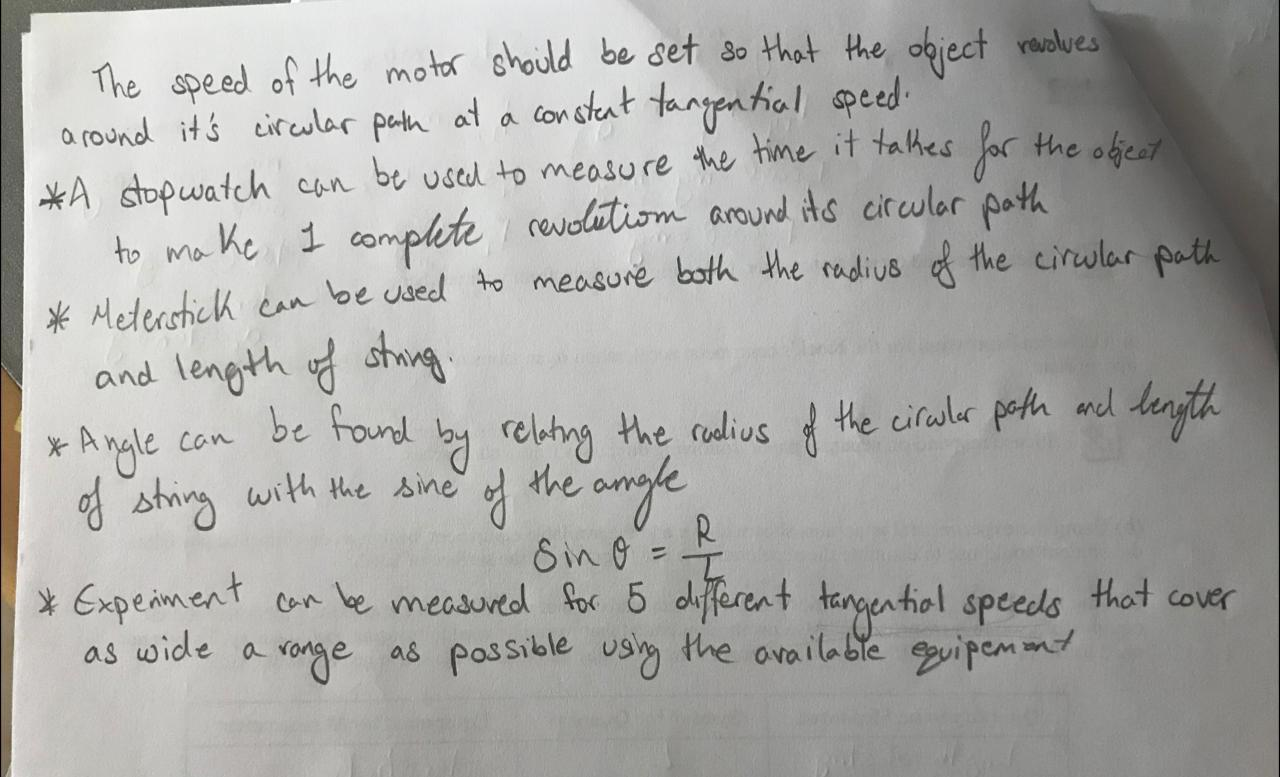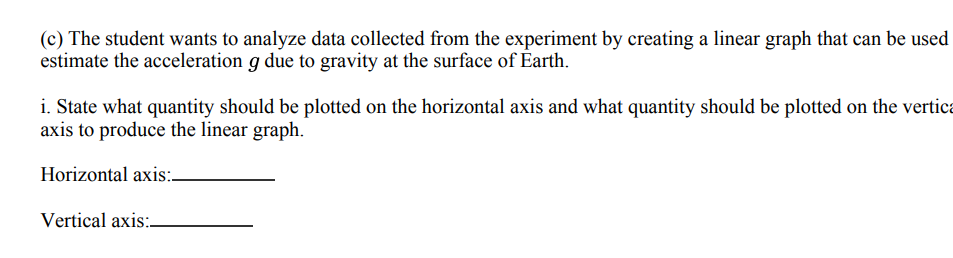Transcribed Image Text: 1. This question is a long free-response question. Show your work for each part of the question. Motor ie L String R M Block In the apparatus shown above, one end of a string of length L is attached to a block of mass M and the other end is connected to the axle of a motor that rotates, causing the block to move in a circle of radius R a constant speed vt such that the string makes an angle with the vertical. A student wants to use the apparatus to make measurements and create a graph that can be used to estimate the acceleration g due to gravity at the surface of Earth. The student can adjust the motor to achieve different tangential speeds of the block. (b) Using the experimental apparatus shown above and the available equipment, design an experimental procedure the student could use to estimate the acceleration g due to gravity at the surface of Earth. i. In the table below, list the quantities and associated symbols that would be measured in the experiment and the equipment used to measure them. Also list the equipment that would be used to measure each quantity. You do not need to fill in every row. If you need additional rows, you may add them to the space just below the table. Quantity to be Measured Symbol for Quantity length of string L radius R Time for block to make I rev. T Equipment for Measurement meter stick meterstick Stopwatch peed ii. Describe an experimental procedure to estimate the acceleration g due to gravity at the surface of Earth using the apparatus. Give enough detail so that another student could replicate the experiment. As needed, include a diagram of the experimental setup. Assume equipment usually found in a school physics laboratory is available. for the object The speed of the motor should be set so that the object revolves around it's circular path at a constant tangential speed *A stopwatch can be used to measure the time it takes to make I complete revolution around its circular path * Meterstick can be used to measure both the radius of the circular path and length of string. * by relating the rodius of the circular path and length of string with the sine of the angle * Experiment can be measured for 5 different tangential speeds that cover Sino- as wide a ronge as possible using the available equipement * Angle can be found R I о (c) The student wants to analyze data collected from the experiment by creating a linear graph that can be used estimate the acceleration g due to gravity at the surface of Earth. i. State what quantity should be plotted on the horizontal axis and what quantity should be plotted on the vertica axis to produce the linear graph. Horizontal axis: Vertical axis: -
More
Transcribed Image Text: 1. This question is a long free-response question. Show your work for each part of the question. Motor ie L String R M Block In the apparatus shown above, one end of a string of length L is attached to a block of mass M and the other end is connected to the axle of a motor that rotates, causing the block to move in a circle of radius R a constant speed vt such that the string makes an angle with the vertical. A student wants to use the apparatus to make measurements and create a graph that can be used to estimate the acceleration g due to gravity at the surface of Earth. The student can adjust the motor to achieve different tangential speeds of the block. (b) Using the experimental apparatus shown above and the available equipment, design an experimental procedure the student could use to estimate the acceleration g due to gravity at the surface of Earth. i. In the table below, list the quantities and associated symbols that would be measured in the experiment and the equipment used to measure them. Also list the equipment that would be used to measure each quantity. You do not need to fill in every row. If you need additional rows, you may add them to the space just below the table. Quantity to be Measured Symbol for Quantity length of string L radius R Time for block to make I rev. T Equipment for Measurement meter stick meterstick Stopwatch peed ii. Describe an experimental procedure to estimate the acceleration g due to gravity at the surface of Earth using the apparatus. Give enough detail so that another student could replicate the experiment. As needed, include a diagram of the experimental setup. Assume equipment usually found in a school physics laboratory is available. for the object The speed of the motor should be set so that the object revolves around it's circular path at a constant tangential speed *A stopwatch can be used to measure the time it takes to make I complete revolution around its circular path * Meterstick can be used to measure both the radius of the circular path and length of string. * by relating the rodius of the circular path and length of string with the sine of the angle * Experiment can be measured for 5 different tangential speeds that cover Sino- as wide a ronge as possible using the available equipement * Angle can be found R I о (c) The student wants to analyze data collected from the experiment by creating a linear graph that can be used estimate the acceleration g due to gravity at the surface of Earth. i. State what quantity should be plotted on the horizontal axis and what quantity should be plotted on the vertica axis to produce the linear graph. Horizontal axis: Vertical axis: -